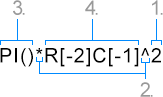Using Formulas in Table Cells

Technical Articles

TX Text Control provides the TableCellFormula property to define formulas for table cells and to display the result of such calculations as cell text. The syntax of those formulas correlates mostly with the formula specification in SpreadsheetML (Reference: Office Open XML File Formats - ISO/IEC 29500 - 1, Chapter: 18.17.2 Syntax) which is also used by most spreadsheet applications such as Microsoft Excel.

A formula can consist of four elements: functions, cell references, constants and operators. The first three represent specific values inside the formula. Operators concatenate these elements and perform the corresponding calculation/operation.

The following example shows a sample formula containing a function, a cell reference, a constant and two operators:1. Constant: 2 is a number constant.

2. Operators: '^' is the caret operator. It exponentiates the value of table cell A2 with 2. The operator '*' multiplies the result of this calculation with Pi.

3. Function: PI() is a function that returns the value of Pi: 3.14159265.

4. Cell Reference: R[-2]C[-1] returns the value of the corresponding table cell (2 rows above and 1 column to the left from the formula's table cell).

Constants

Operators

Functions

Cell References